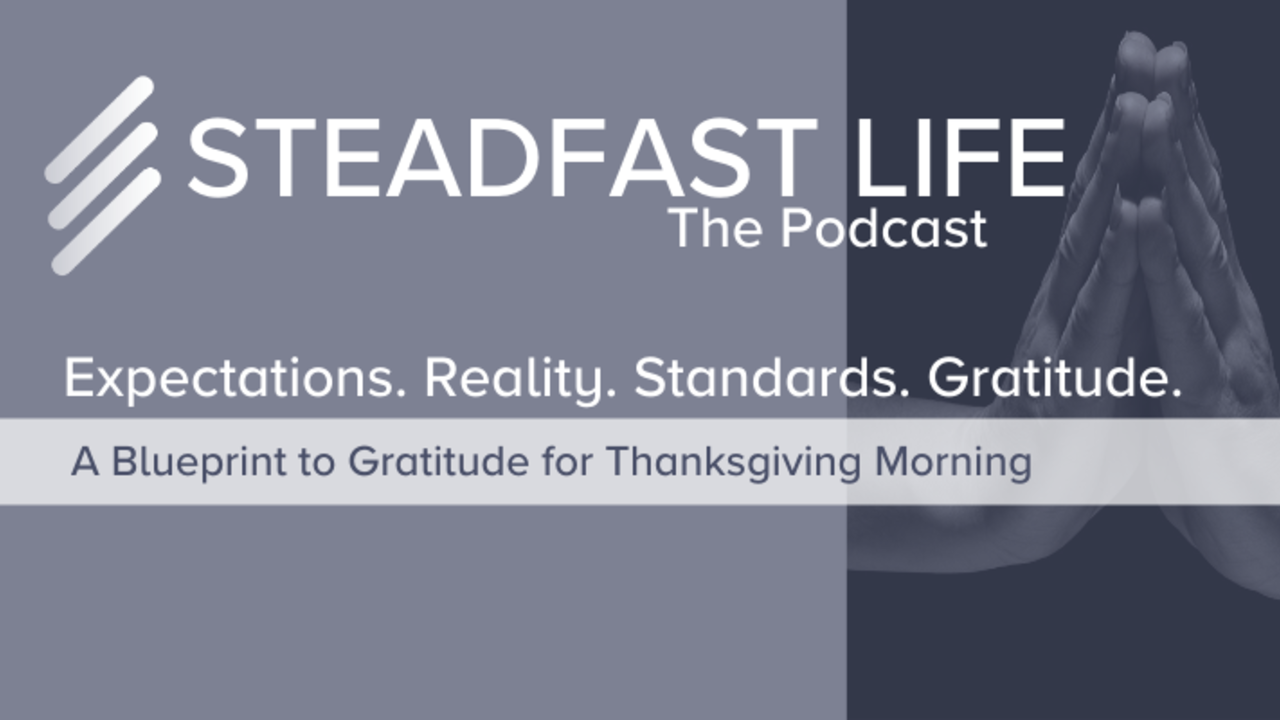# Expectations. Reality. Standards. Gratitude

Uncategorized Nov 28, 2019I promise this isn't your run of the mill "count your blessings" take on gratitude.  The question is, when reality and your expectations do not align, which do blame for your missing gratitude?

For my math people...

• R(x) << E(x) = Tragedy
• R(x) < E(x)   = Bummer
• R(x) = E(x)   = Whatever
• R(x) > E(x)   = Rad
• R(x) >> E(x) = Miracle

Where:

• R(x) = Value of reality at event, x
• E(x) = Value of Expectations at event, x